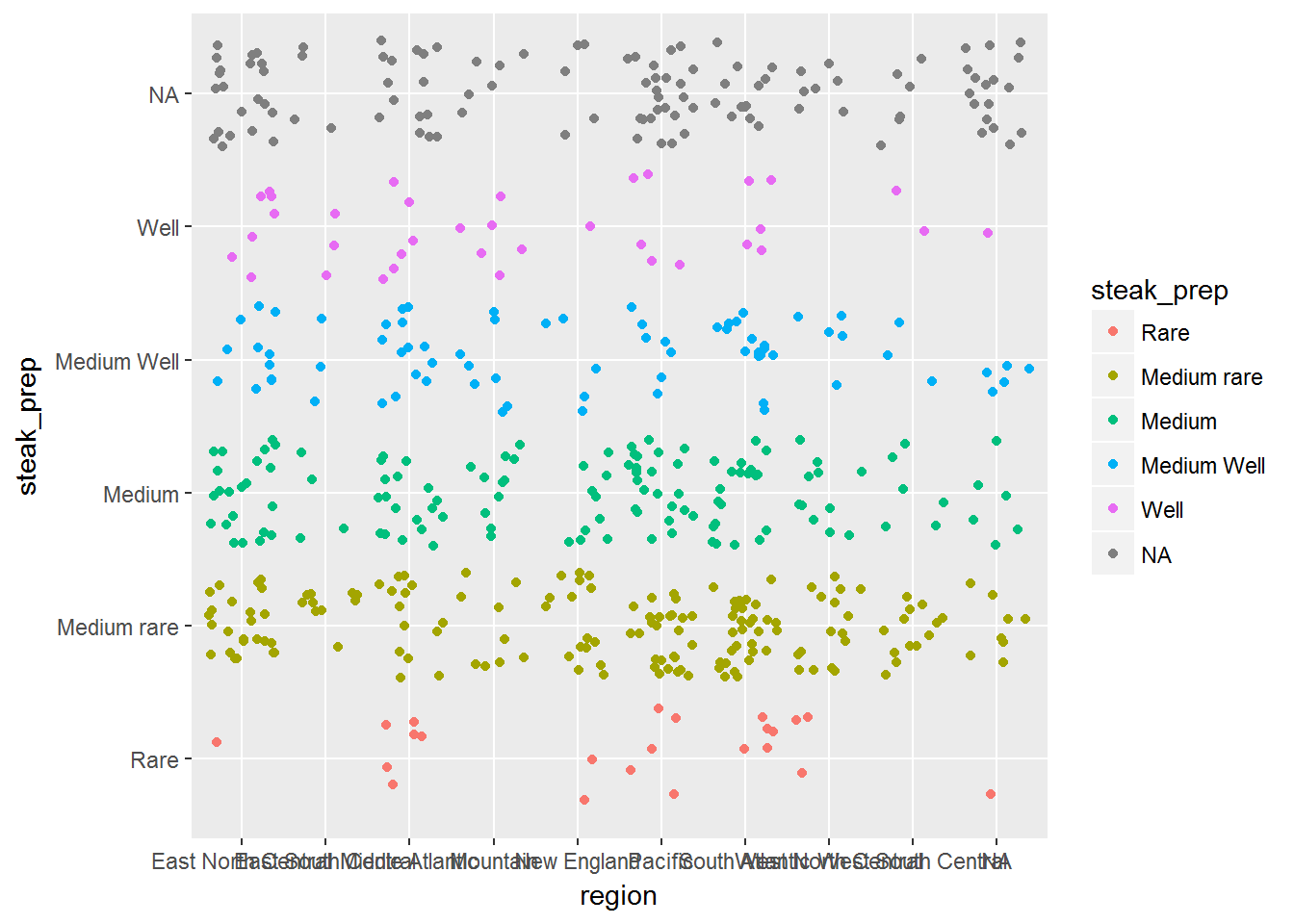# How does `+` in ggplot work?

I am a big fan of `ggplot2`. As one of the most popular R packages, I’m clearly not alone. As well as making great plots, it’s given me a deeper concept of what a plot is, and to use ggplot terms, how data maps to a geom.

One of the most recognisable parts of the package is the concept of adding layers to plots. We’ll use the `steak_survey` dataset from the `fivethirtyeight` package. (Check out their website for some interesting data related stories!)

As shown below, the code to create a plot literally adds layers, using `+`. But how does the `+` work? We’re not adding numbers.. so what magic is going on? It seems so intuitive that it’s only on a second glance do we realise that this isn’t usual behaviour!

``````library(ggplot2)
library(fivethirtyeight)

steak <- fivethirtyeight::steak_survey

# Create example plot

ggplot(steak, aes(region, steak_prep, col = steak_prep)) + geom_jitter() + coord_flip()``````To get our answer, there are two parts to understand.

## Part One - What exactly is `+`?

It seems pretty obvious. Everyone will recognise the `+` symbol from an early age. But what is it exactly in R?

``1 + 2``
``##  3``

`+` is in fact, just another function. The ability to perform `x + y` is syntactic sugar for the language. It’s much easier than typing it’s functional form, shown in the example below.

```+`(1, 2)``
``##  3``
``class(`+`)``
``##  "function"``

So when we call `ggplot() + geom_jitter()`, `+` is acting as a function like any other. We could recreate our above graph with the code below, as it’s equivalent (apart from the missing `coord_flip`. Sorry about those titles!).

```+`(ggplot(steak, aes(region, steak_prep, col = steak_prep)), geom_jitter())``So that explains what `+` is, but how does `R` know what the sum of `ggplot() + geom_jitter` is?

Well, onto the next part!

## Part Two - Generic Functions

To get out answer, we need to take a small detour.

Let’s take a look at `print()`

``print("abcdef") # works as expected``
``##  "abcdef"``
``print(123) # Still works as expected``
``##  123``

Not very interesting so far, so let’s break it.

``````time <- Sys.time() # return current time
typeof(time) # double? But it's a date-time!?``````
``##  "double"``
``print(time) # This prints the date though..``
``##  "2018-04-08 20:15:25 BST"``

As you can see, the time, is not strictly a time. It’s actually a double, counting the number of seconds since the Unix epoch. But when we print it, it returns a pretty date? Very odd.

``print(as.numeric(time)) # That's what it actually is``
``##  1523214926``

So how does it know to print something else? The answer- Generic functions.

When you call print, a bit more goes on behind the scenes than you might initially realise. `print` is known as a generic method. Depending on the class of the object is it trying to print, a different print function will be called! You can see all of the methods for print using the `methods()` function.

``typeof(print)``
``##  "closure"``
``class(print)``
``##  "function"``
``head(methods(print))``
``````##  "print.acf"     "print.AES"     "print.anova"   "print.aov"
##  "print.aovlist" "print.ar"``````
``length(methods(print))``
``##  227``

When you print the time, it checks to see the class of the object. As our time object has the classes of `POSIXct` and `POSIXt`. It searches for the `print.POSIXct()` method, to nicely format out time. If it couldn’t find a `print.POSIXct()` method, it would look at the next class, `POSIXt`. If it still cannot find a method, it will use the `print.default()` method.

### But what does this have to do with `+`?

You may have already made the leap, but as we discussed earlier, `+` is just another function. As such, we could assign it another method to it! In this case, it’s as below.

``methods(`+`)``
``````##  +.Date   +.gg*    +.POSIXt
## see '?methods' for accessing help and source code``````
``# Note +.gg* - This is the method we are after!``

What exactly it does is the content of another post.

In summary, `+()` is a function. `ggplot2` cleverly defines a new method for `+()`, which is then uses to allow the layering ability that we have in ggplot!

1. The help page for the ggplot `+` method.
2. For more about generic functions, check out Advanced R, by Hadley Wickham, who also authored the `ggplot` package!